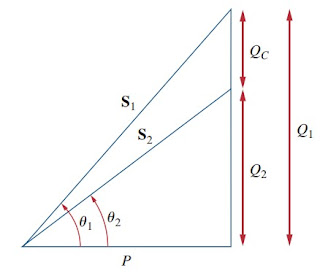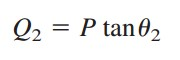omG8HI9LSKFW6lhCj8prTs0Z6lUhdOT9Jhi1Sf4m

# What is Power Factor Correction for AC Circuits

Most domestic loads (such as washing machines, air conditioners, and refrigerators) and industrial loads (such as induction motors) are inductive and operate at a low lagging power factor. Although the inductive nature of the load cannot be changed, we can increase its power factor.
The process of increasing the power factor without altering the voltage or current to the original load is known as power factor correction.
Make sure to read what is ac circuit first.

## Power Factor Correction

Since most loads are inductive, as shown in Figure.(1a), a load's power factor is improved or corrected by deliberately installing a capacitor in parallel with a load, as shown in Figure.(1b). The effect of adding the capacitor can be illustrated using either the power triangle or the phasor diagram of the currents involved.

Figure.(2) shows the latter, where it is assumed that the circuit in Figure.(1a) has a power factor of cosθ1, while the one in Figure.(1b) has a power factor of cosθ2. It is evident from Figure.(2) that adding the capacitor has caused the phase angle between the supplied voltage and current to reduce from θ1 to θ2, thereby increasing the power factor.Figure 1. Power factor correction : (a) original inductive load, (b) inductive load with improved power factor
We also notice from the magnitudes of the vectors in Figure.(2) that with the same supplied voltage, the circuit in Figure.(1a) draws larger current IL than the current I was drawn by the circuit in Figure.(1b). Power companies charge more for larger currents because they result in increased power losses (by a squared factor, since P = IL2R).Figure 2. Phasor diagram showing the effect of adding a capacitor in parallel with the inductive load
Therefore, it is beneficial to both the power company and the consumer that every effort is made to minimize the current level or keep the power factor as close to unity as possible. By choosing a suitable size for the capacitor, the current can be made to be completely in phase with the voltage, implying unity power factor.

We can look at the power factor correction from another perspective. Consider the power triangle in Figure.(3).Figure 3. Power triangle illustrating power factor correction
If the original inductive load has apparent power S1, then(1)
If we desire to increase the power factor from cosθ1 to cosθ2 without altering the real power (i.e., P = S2 cosθ2), then the new reactive power is(2)
The reduction in the reactive power is caused by the shunt capacitor; that is(3)
QC = Vrms2/XC = ωCVrms2. The value of the required shunt capacitance C is determined as(4)
Note that the real power P dissipated by the load is not affected by the power factor correction because the average power due to the capaci- tance is zero.

Although the most common situation in practice is that of an inductive load, it is also possible that the load is capacitive; that is, the load is operating at a leading power factor. In this case, an inductor should be connected across the load for power factor correction. The required shunt inductance L can be calculated from(5)
where QL = Q1 - Q2, the difference between the new and old reactive powers.

## Power Factor Correction Examples

When connected to a 120-V (rms), 60-Hz power line, a load absorbs 4 kW at a lagging power factor of 0.8. Find the value of capacitance necessary to raise the pf to 0.95.
Solution :
If the pf = 0.8, then
where θ1 is the phase difference between voltage and current. We obtain the apparent power from the real power and the pf as
The reactive power is
When the pf is raised to 0.95,
The real power P has not changed. But the apparent power has changed; its new value is
The new reactive power is
The difference between the new and old reactive powers is due to the parallel addition of the capacitor to the load. The reactive power due to the capacitor is
and
Note: Capacitors are normally purchased for voltages they expect to see. In this case, the maximum voltage this capacitor will see is about 170 V peak. We would suggest purchasing a capacitor with a voltage rating equal to, say, 200 V.

Have you understood what is power factor correction? Don't forget to share and subscribe! Happy learning!
Reference:  Fundamentals of electric circuits by Charles K. Alexander and Matthew N. O. Sadiku.
Related Posts
SHARE